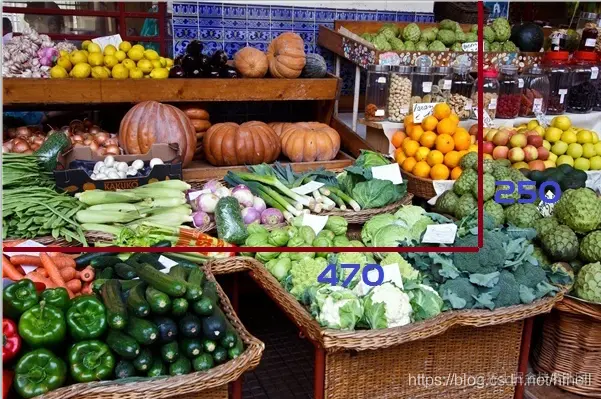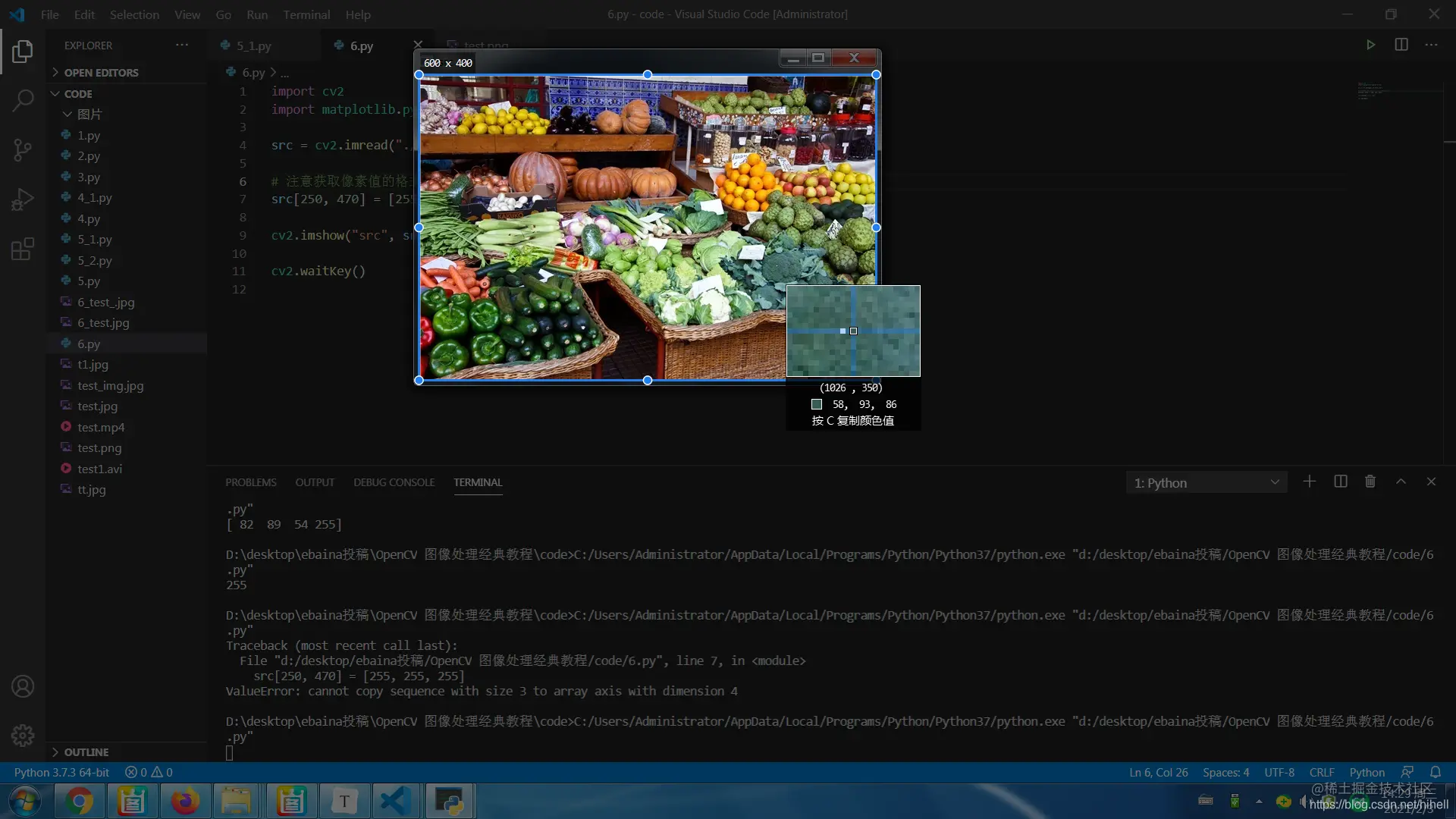# Fanwai 6 Different operations for image details in Python opencv

2022-01-30 21:39:32

「 This is my participation 11 The fourth of the yuegengwen challenge 2 God , Check out the activity details ：2021 One last more challenge

## The way this series is written

This series of column writing will adopt the original question and answer writing form , Quickly let you learn OpenCV My primary 、 intermediate 、 Advanced knowledge .

## 6. stay Python OpenCV Different operations for image details

The goal of this blog is to explain the disassembly of an image , Including the description of image pixels , Acquisition and modification of image attribute information , Image target area ROI Related content , And knowledge of image channels （ Including split channel and merge channel ）

These contents are different from `numpy` The library is very close , If from the perspective of learning , I suggest you reserve `numpy` Related knowledge .

### Read and modify the pixel value of the image

In a previous blog post , We have learned how to read an image , Use `cv2.imread` Function , And master the two key parameters of the function .

After reading the picture , We can directly use the operation array to obtain the color at any position of the image , Generally, the default order of this color is BGR.

The test code is as follows ：

``````import cv2
import matplotlib.pyplot as plt

#  obtain  100 x 100  Pixel value of the position
print(src[100, 100])

cv2.imshow("src", src)

cv2.waitKey()
Copy code ``````

Here, first get 100 x 100 Pixel value of the position . `src[100,100]` You get three values , Respectively corresponding BGR The value of the channel . We mark a pixel on the picture ,rows = 250,cols=470 , Next, modify the above code , See what you get BGR value .

``````import cv2
import matplotlib.pyplot as plt

#  Note that the format for obtaining pixel values is  [cols,rows]
print(src[250, 470])

cv2.imshow("src", src)

cv2.waitKey()
Copy code ``````The special attention above is , The format for obtaining pixel values is `[cols,rows]`, At the top of the list , Line after .

What we got above is BGR value , You can also get only the value of a single channel , The corresponding code is `[cols,rows,channel]`, Corresponding to the code section , As shown below ：

``````#  Get the blue channel value
print(src[250, 470, 0])
Copy code ``````

The blue channel corresponds to 0, The green channel is 1, The red channel is 2, The above three values are exceeded , There will be the following mistakes ：

``````IndexError: index 3 is out of bounds for axis 2 with size 3
Copy code ``````

At present, if you read the gray image directly , For example, the following code , The values of the three channels are the same .

``````import cv2
import matplotlib.pyplot as plt

#  Note that the format for obtaining pixel values is  [cols,rows]
print(src[250, 470])

cv2.imshow("src", src)

cv2.waitKey()
Copy code ``````

There is also a potential coding problem in this place , If you read a four channel picture , That is, the picture has transparency , The index value of the array can be read 3, That is, the following code is correct .

``````import cv2
import matplotlib.pyplot as plt

#  Note that the format for obtaining pixel values is  [cols,rows]
print(src[250, 470, 3])

cv2.imshow("src", src)

cv2.waitKey()
Copy code ``````

`src[250, 470, 3]` Successfully read the value of the transparent channel .

We can modify the value for specific pixels , For example, the following code

``````import cv2
import matplotlib.pyplot as plt

#  Note that the format for obtaining pixel values is  [cols,rows]
src[250, 470] = [255, 255, 255]

cv2.imshow("src", src)

cv2.waitKey()
Copy code ``````

Note the position pointed by the red arrow in the figure below , A white bright spot appears , Use this method , Can make a 【 Pepper and salt picture 】.There is a potential need to pay attention to this place BUG, Number of channels to read pictures , Determines the number of array elements you copy , For example, the following code will report an error .

``````import cv2
import matplotlib.pyplot as plt

#  Note that the format for obtaining pixel values is  [cols,rows]
src[250, 470] = [255, 255, 255, 255]

cv2.imshow("src", src)

cv2.waitKey()
Copy code ``````

The error messages are similar , Prompt that the array dimensions are different .

``````ValueError: cannot copy sequence with size 4 to array axis with dimension 3
Copy code ``````

Finally, operate the pixels of the image in the above way , It's very time consuming , Because the pixel data of a picture is very large , Generally, it can be used numpy Integrated approach , Don't use this most clumsy way .

Use numpy Get channel value , Note that this method gets scalar , If you want to get all BGR Value , You need to use `array.item()` Get... In turn .

``````import cv2
import numpy as np

print(src[100, 100])
b = src.item(100, 100, 0)
g = src.item(100, 100, 1)
r = src.item(100, 100, 2)
print(b, g, r)

cv2.imshow("src", src)
cv2.waitKey()
Copy code ``````

If you want to set this value , Use it directly `itemset` Function .

``````src.itemset((100, 100, 0), 200)
print(src[100, 100])
Copy code ``````

You can find any picture for the corresponding test , The operation effect is as follows ：

``````[ 31  68 118]
31 68 118
[200  68 118]
Copy code ``````

### OpenCV Analysis of common problems of image attributes in

For an image , In addition to the pixel matrix , There is also a very important content , Is the attribute of the image , These include lines 、 Column 、 passageway 、 data type , Number of pixels 、 Image, shape, etc .

for example , We use it a lot `img.shape` To get the shape of the image , In particular , The returned content is the number of rows （rows）, Number of columns （cols）, And the number of channels （channels）, And the return value type is a tuple .

If you read the image , Set tight read grayscale , That will only return the number of rows and columns , Accordingly, through this value, it is easy to determine the type of image you load .

For example, the following code , Read the same picture in different ways , Different shapes of the output image .

``````import cv2
import numpy as np

#  Select a  png  picture , Different channels can be read
#  Four channels , Contains transparent channels
print(src1.shape)
#  grayscale
print(src2.shape)
#  Three channels
print(src3.shape)
Copy code ``````

The output result can quickly read out whether the image is a color image or a gray image .

``````(397, 595, 4)
(397, 595)
(397, 595, 3)
Copy code ``````

Use `img.size` You can quickly return the total number of pixels in the image , The test code is as follows ：

``````#  Select a  png  picture , Different channels can be read
#  Four channels , Contains transparent channels
print(src1.shape)
print(src1.size)
#  grayscale
print(src2.shape)
print(src2.size)
#  Three channels
print(src3.shape)
print(src3.size)
Copy code ``````

We still have three different reading methods , The number of pixels read is as follows ：

``````(397, 595, 4)
944860
(397, 595)
236215
(397, 595, 3)
708645
Copy code ``````

Be careful , The number of pixels of gray image and color image is different , They had the following relationship before .

The number of pixels in a grayscale image = Row number x Number of columns = 397 x 595 = 236215

The number of pixels in a color image = Row number x Number of columns x The channel number = 944860 （ Four channels ）/ 708645（ Three channels ）

Use `img.dtype` Property to get the type of image , As follows ：

``````print(src1.dtype)
Copy code ``````

The value read here , It's all the same `uint8` Express 8 Bit image , Here you can remember that as long as it is `uint8` Format , That corresponds to BGR The range of values is in `[0,255] ` Between .

When operating the above attribute values , The following will appear BUG, The BUG The general solution is to check whether the picture is read normally , You need to pay special attention to ：

``````AttributeError: 'NoneType' object has no attribute 'shape'
Copy code ``````
``````print(src1.dtype)
Copy code ``````

The value read here , It's all the same `uint8` Express 8 Bit image , Here you can remember that as long as it is `uint8` Format , That corresponds to BGR The range of values is in `[0,255] ` Between .

When operating the above attribute values , The following will appear BUG, The BUG The general solution is to check whether the picture is read normally , You need to pay special attention to ：

``````AttributeError: 'NoneType' object has no attribute 'shape'
Copy code ``````